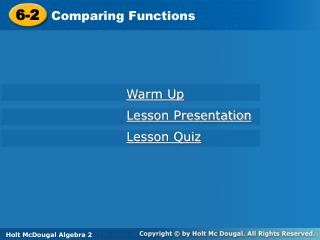Download Presentation6-2# 6-2 - PowerPoint PPT Presentation

Download Presentation##### 6-2

Download Policy: Content on the Website is provided to you AS IS for your information and personal use and may not be sold / licensed / shared on other websites without getting consent from its author. While downloading, if for some reason you are not able to download a presentation, the publisher may have deleted the file from their server.

- - - - - - - - - - - - - - - - - - - - - - - - - - - E N D - - - - - - - - - - - - - - - - - - - - - - - - - - -
##### Presentation Transcript

1. 6-2 Comparing Functions Warm Up Lesson Presentation Lesson Quiz Holt McDougal Algebra 2 Holt Algebra 2

3. Warm Up For each function, determine whether the graph opens upward or downward. downward 1. f(x) = -4x2 + 6x + 1 upward 2. f(x) = 8x2 – x - 2 Write each function in slope-intercept form. y = -3x + 10 3. y + 3x =10 4. -6y – 12x = 24 y = -2x - 4

4. Objectives Estimate and compare rates of change.

5. The graph of the exponential function y=0.2491e0.0081x approximates the population growth in Baltimore, Maryland.

6. The graph of the exponential function y=0.0023e0.0089x approximates the population growth in Hagerstown, Maryland. The trends can be used to predict what the population will be in the future in each city. In this lesson you will compare the graphs of linear, quadratic and exponential functions.

7. Example 1: Comparing the Average Rates of Change of Two Functions. George tracked the cost of gas from two separate gas stations. The table shows the cost of gas for one of the stations and the graph shows the cost of gas for the second station. Compare the average rates and explain what the difference in rate of change represents.

8. The rate of change for Gas Station A is about 3.0. The rate of change for Gas Station B is about 2.9. The rate of change is the cost per gallon for each of the Stations. The cost is less at Gas Station B.

9. Check It Out! Example 1 John and Mike opened savings accounts on the same day. They did not deposit any money initially, but deposited each week as shown by the graph and the table. Compare the average rates of change and explain what the rates represent in this situation.

10. Mike’s average rate of change ism = 124 -20 = 104 = 26 5-1 4 John’s average rate of change ism = 204 -25 = 179 ≈25.57 8-1 7 Example 1 continued Mike’s average rate of change is 26. John’s average rate of change is ≈ 25.57. The rate of change is the average amount of money saved per week. In this case, Mike’s rate of change is larger than John’s, so he saves about \$0.43 more than John per week

11. Helpful Hint Remember to find the average rate of change over a data set, find the slope between the first and last data point. Example 1 continued

12. Find the Average Rate of Change from x=0 to x=2 a‹b When x=0, y=4 and when x=2, y=-3 so average rate of change is -7 for this interval

13. Compare the equation , the graph, and the table. Which has the steepest rate of change from x=1 to x=2. What is the value? Equation: (1,5)(2,2) Avg. Rate of Chg.=-3 (1,2)(2,0) Avg. Rate of Chg.=-2 (1,0) (2,-1) Avg. Rate of Chg.=-1

14. You Try • Which function has a greater rate of change from x=0 to x=1? • Which function has a greater rate of change from x=2 and x=3? • Does g(x) ever have a greater rate of change than f(x)?

15. Use graphing to find the solution to the system of equations

16. Factor (7x+6i)(7x-6i)

17. You Try: Factor

18. Assignment: Worksheet